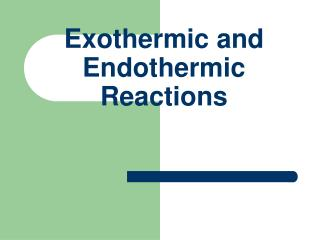DownloadDownload PresentationExothermic and Endothermic Reactions

# Exothermic and Endothermic Reactions

Download Presentation## Exothermic and Endothermic Reactions

- - - - - - - - - - - - - - - - - - - - - - - - - - - E N D - - - - - - - - - - - - - - - - - - - - - - - - - - -
##### Presentation Transcript

1. Exothermic and Endothermic Reactions

2. Chemical Reactions • All chemical reactions involve bond breaking and bond forming. • Energy is needed to break bonds and released when bonds are formed. • Chemical reactions are accompanied by a change in energy, mainly in the form of heat.

3. Exothermic and Endothermic Reactions • A reaction in which heat is given out is exothermic. • A reaction in which heat is taken in is endothermic.

4. Combustion of Hydrocarbons • When hydrocarbons are burned carbon dioxide, water vapour and large amounts of heat are produced. • Hydrocarbons (particularly alkanes) are excellent fuels e.g. methane, propane, butane and petrol.

5. Heat of Reaction • The heat of reaction, ΔH, of a chemical reaction is the heat in kilojoules released or absorbed when the number of moles of reactants indicated, in the balanced equation describing the reaction, react completely. • For an exothermic reaction ΔH is negative(-) • For an endothermic reaction ΔH is positive(+)

6. Bond Energy • Bond energy is the amount of energy in kilojoules needed to break one mole of bonds of the same type, all species being in the gaseous state. • The average C-H bond energy in methane is 412kj mol-1 i.e. E(C-H) = 412kj mol-1 . • The energy of a particular bond type can vary. • It is usual to quote the average bond energies.

7. Heat of Combustion • The heat of combustion of a substance is the heat change in kilojoules when one mole of the substance is completely burned in excess oxygen.

8. Bomb Calorimeter • Used to find accurate values for heats of combustion. • Sample is ignited using an electrically heated wire. • Excess oxygen ensures complete combustion of the sample. • Heat produced by the rapid and complete combustion of the sample heats the water and the temperature is recorded.

9. Bomb Calorimeter

10. Bomb Calorimeter • The apparatus is well insulated to prevent heat loss. • The amount of heat used to start burning can be calculated and removed.

11. Heat of Combustion of Different Fuels • Methane (natural gas) -890 kj mol-1 • Propane (LPG) -2219 kj mol-1 • Hydrogen -286 kj mol-1 • Petrol (octane) -5470 kj mol-1

12. Heat of Formation • The heat of formation is the heat change in kilojoules, when one mole of a substance is formed from its elements in their standard state.

13. Law of Conservation of Energy • The law of conservation of energy states that energy cannot be created or destroyed, but only changed from one form to another.

14. Hess’s Law • Hess’s Law states that the heat change of a reaction depends only on the initial and final states of the reaction and is independent of the route by which the reaction may occur. • for a reaction described by a given equation, the heat of reaction equals the sum of the heats of formation of the products of the reaction less the sum of the heats of formation of the reactants of the reaction. • ΔHr = ΔHf (products) -ΔHf (reactants)